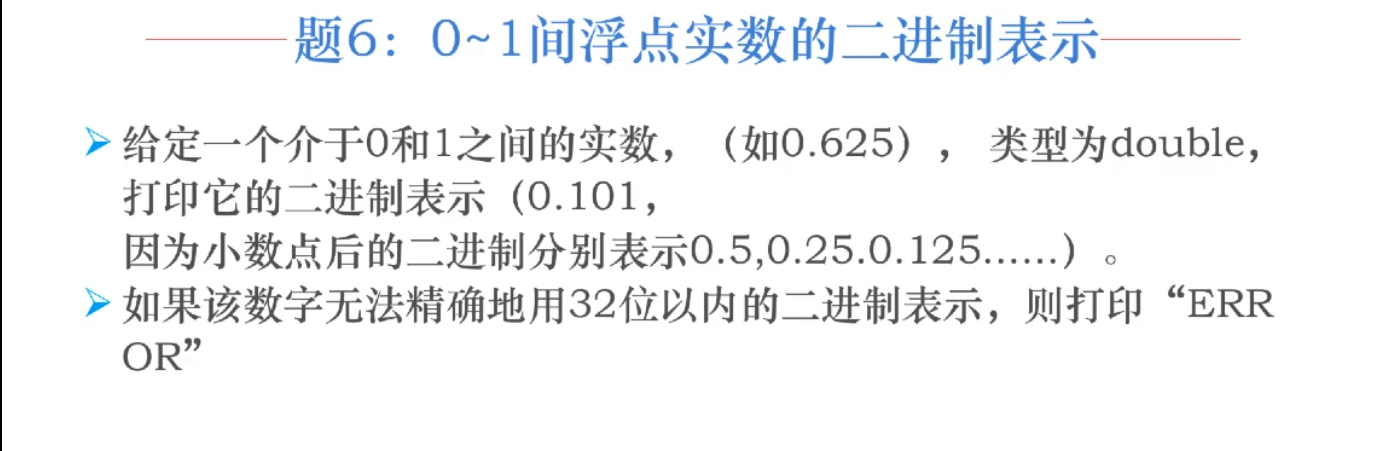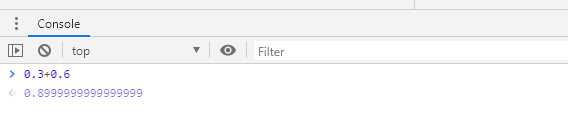• 二进制表示浮点数Prerequisite: Number systems 先决条件： 数字系统 We all very well know that very small and very large numbers in the decimal number system are represented using scientific notation ...

二进制表示浮点数
Prerequisite: Number systems
先决条件： 数字系统
We all very well know that very small and very large numbers in the decimal number system are represented using scientific notation form by stating a number (mantissa) and an exponent in the power of 10. Some of the examples are 6.27 * 10-27 and 5.21 * 1034. Similarly, Binary numbers can also be represented in the same form by stating a number (mantissa) and an exponent of 2. The format of this representation will be different for different machines.
众所周知，十进制数系统中的非常小和非常大的数字都是用科学计数形式表示的，即数字( 尾数 )和幂为10的指数。 一些示例是6.27 * 10 -27和5.21 * 10 34 。 类似地，二进制数字也可以通过表示数字( 尾数 )和2的指数来以相同的形式表示。 对于不同的机器，此表示形式的格式将有所不同。
The 16-bit machine consists of 10 bits as the mantissa and 6 bits for the exponent part whereas 24-bit machine consists of 15 bits for mantissa and 9 bits for exponent.
16位机器由10位尾数组成， 指数部分为6位，而24位机器由尾数15位组成， 指数部分为9位。
Format of the 16-bit machine can be represented as:
16位计算机的格式可以表示为：

Mantissa PartExponent Part0110011010101010
尾数部分  指数部分  0110011010  101010

The mantissa is written in 2's complement form, so the MSB of the Mantissa can be thought of as a sign bit. The binary point is assumed to be to the right of this sign bit. The 6-bit of the exponent can be used to represent 0 to 63, however, to express negative exponents a number (32)10 or (100000)2 is added to the desired exponent.
尾数以2的补码形式编写，因此可以将尾数的MSB视为符号位。 假定二进制点在该符号位的右边。 指数的6位可用于表示0到63 ，但是，要表示负指数，则将数字(32) 10或(100000) 2添加到所需的指数。
Excess-32 Representation: This is a common system to represent floating-point numbers. In this notation, to represent a negative exponent, we add (32)10 to the given exponent which are given by the 6 bits.
Excess-32表示法 ：这是表示浮点数的通用系统。 在这种表示法中，为了表示负指数，我们将(32) 10加到由6位给出的给定指数上。
Given table illustrates representation of exponent part.
给定的表说明了指数部分的表示。

Desired Exponent2's complement notationExcess-32 notation (in 6 bits)Binary representation-32100000100000 +100000 = 000000000000-31100001100001 +100000 = 000001000001-30100010100010 +100000 = 000010000010-15110001110001 +100000 = 010001010001 0000000000000 +100000 = 100000100000 +1000001000001 +100000 = 100001100001+15001111001111 +100000 = 101111101111+30011110011110 +100000 = 111110111110+31011111011111 +100000 = 111111111111
期望指数  2的补码表示法  多余的32位表示法(6位)  二进制表示  -32  100000  100000 +100000 = 000000  000000  -31  100001  100001 +100000 = 000001  000001  -30  100010  100010 +100000 = 000010  000010  -15  110001  110001 +100000 = 010001  010001  0  000000  000000 +100000 = 100000  100000  +1  000001  000001 +100000 = 100001  100001  +15  001111  001111 +100000 = 101111  101111  +30  011110  011110 +100000 = 111110  111110  +31  011111  011111 +100000 = 111111  111111

Mantissa PartExponent Part0110011010101010
尾数部分  指数部分  0110011010  101010

As given above, the floating-point number given in the above format is:
如上所述，以上述格式给出的浮点数为：
At the extreme left (MSB) is the sign-bit '0', which represents it is a positive number. Also, just after the sign-bit, we assume a binary point. Thus,
最左端( MSB )是符号位“ 0” ，表示它是一个正数。 同样，在符号位之后，我们假设一个二进制点。 从而，
    In Mantissa Part: .110011010
Subtracting 100000   001010 (i.e.,10 in decimal, so exponent part is 210)
The number is N
= +(.110011010)2 * 210
= +(1100110100.00)
= +(820)10


Example 1: Express the following decimal number into 16-bit floating point number (45365.125)10
示例1：将以下十进制数表示为16位浮点数(45365.125) 10
Solution:
解：
    Binary equivalent of (45365.125)10: 1011000100110101.001
Binary format: .1011000100110101 * 216
Mantissa: + .101100010
Exponent: 010000 (Value of exponent is 16)
Equivalent exponent: 010000 + 100000 = 110000


Since the number is a positive number an additional sign-bit '0' is added in the MSB.
由于该数字为正数，因此在MSB中添加了一个附加符号位“ 0”。
So, the floating-point format will be 0101100010110000
因此，浮点格式将为0101100010110000
Example 2: What floating point number do the given number 0100101001101011 represents?
示例2：给定的数字0100101001101011代表什么浮点数？
Solution:
解：
At the extreme left (MSB) is the sign-bit '0' which represents it is a positive number. Also, just after the sign-bit we assume a binary point. Thus,
最左边(MSB)是符号位“ 0”，表示它是一个正数。 同样，在符号位之后，我们假设一个二进制点。 从而，
    In Mantissa Part: .100101001
Subtracting 100000 001011 (i.e.,11 in decimal, so exponent part is 211)
The number is N
= +(.100101001)2 * 211
= +(10010100100.0)
= +(1188)10


翻译自: https://www.includehelp.com/basics/floating-point-representation-of-binary-numbers.aspx

二进制表示浮点数

展开全文补码 python openid ggplot2
• 5. 二进制表示浮点数 问题 给定一个介于0和1之间的实数(如0.625)，类型为double,打印它的二进制表示(0.101,因为小数点后的二进制分别表示2^(-1), 2^(-2), 2^(-3)...) 如果该数字无法精确地用32位以内的二进制表示，...
Chapter1: 位运算的奇技淫巧
5. 二进制表示浮点数
问题
给定一个介于0和1之间的实数(如0.625)，类型为double,打印它的二进制表示(0.101,因为小数点后的二进制分别表示2^(-1), 2^(-2), 2^(-3)...)
如果该数字无法精确地用32位以内的二进制表示，则打印"ERROR"
算法
感觉这题跟位运算关系不大，就是按照用二进制表示浮点数的算法，用字符串拼接的方法进行编程
思路：将该浮点数 *2 得到一个新的数 num,如果 num > 1 则在0. 后拼接上1, 否则拼接上 0, 循环直至 num==0
string binaryDouble(double num){
string s="0.";//#include<string>
while(num!=0){
num*=2;
if(num>=1){
s+="1";
num-=1;
}
else{
s+="0";
}
if(s.length()==34){
return "ERROR";
}
}

return s;

}

参考资料
 二进制表示浮点数
 C++字符串
展开全文• public class 二进制表示浮点数 { /** * @param args */ public static void main(String[] args) { double num = 0.625; //拼接字符串 StringBuilder sb = new StringBuilder("0."); while (num >...
题目如下:public class 二进制表示浮点数 {

/**
* @param args
*/
public static void main(String[] args) {
double num = 0.625;
//拼接字符串
StringBuilder sb = new StringBuilder("0.");
while (num > 0) {
//乘以2得出整数部分
double r = num*2;
//判断整数部分
if (r>=1) {
sb.append("1");
//消除整数部分
num = r-1;
}else {
sb.append("0");
num = r;
}
//带上了0.所以长度是34
if (sb.length()>34) {
System.out.println("ERROR");
return;
}
}

System.out.println(sb.toString());

}

}


展开全文算法 java
• 二进制存储浮点数不精确问题欢迎使用Markdown编辑器新的改变功能快捷键合理的创建标题，有助于目录的生成如何改变文本的样式插入链接与图片如何插入一段漂亮的代码片生成一个适合你的列表创建一个表格设定内容居中、...
二进制存储浮点数不精确问题
项目中如果涉及金额等精确浮点数的存储，一般会要求使用BigDecimal类型去存储，而不是使用Double或者Float等默认精度类型存储，那为什么会这么要求，大部分都知道：因为二进制对于小数的存储有可能会发生精度丢失。那具体原因呢？
计算机如何存储数字
首先是整数： 整数在计算机中的存储是以补码的方式存储的。整数的补码就是它本身，负数的补码是它除了符号位的位数取反加1.（之所以使用补码是为了简化计算机基本运算电路，使加减法都只需要用加法电路实现，用加法代替减法） 存储范围是：-2n-1 ~ 2n-1 - 1,n是存储空间，如计算机是32位，那n就是32
举例：
十进制原码反码补码13000011010000110100001101-710000111111110001111001
计算方式： 十进制到源码：除二取余，然后倒序排列，高位补零（负数首位为1） 原码：就是符号加绝对值 反码：正数的反码就是原码，负数的反码是符号位不变，其他位取反 补码：正数的补码就是原码，负数的补码有两种计算方式，一是反码+1，二是原码从右往左找第一个1，1的右边（包含1）不变，1的左边全部取反（不包括符号位） 也就是说正数原码=反码=补码、负数补码=反码+1 注意：二进制补码表示法只有一个0，也就是（0000）2，-0统一表示成2n-1，也就是（1000）2= -8
然后是小数的计算方式： 浮点数是计算机用于表示实数的一种方法，类似于科学计算法，如：
314.4=3.144x1020.00314=3.14x10-3 那带小数的实数如何使用二进制表示？ 首先是整数部分，整数部分跟单个整数的转换没有区别，照样除二取余，然后倒序排列，高位补零（负数首位为1）得到实数的整数部分的二进制，先放在这。接下来是小数部分，步骤为： 对小数部分乘以2，得到结果 取结果的整数位（0或1），如果结果还有小数位，则再拿小数部分乘以2，再取整数部分，以此类推，直到小数部分为0或者位数已经达到了计算机存储上限
浮点数为何会精度丢失
来看个例子，对于python或者js这样的语言来说，一个简单的浮点计算都有可能出错，比如0.3+0.6，在js中结果是什么？我们可以看到，这么简单的计算，居然得不到正确的0.9的结果，而是无限循环的小数。那么原因是什么呢？如果上边的内容你已经看懂了，那么这个问题就很好回答了。 计算机存储小数的时候，由于计算机制的原因，很有可能存储不到精确的数值，而是一个近似数，拿0.3举例来说：
0.3x2=0.6，得到第一位是00.6x2=1.2，得到第二位是10.2x2=0.4，得到第三位是00.4x2=0.8，得到第四位是00.8x2=1.6，得到第五位是1小数位又得到了0.6，回到了步骤2，永远也执行不完 一直执行上述的步骤，直到生成的位数已经达到了计算机上限，有可能是32位也有可能是64位，后边的内容就被抛弃掉了。 只有像0.5这样的能够准备被表示为2-1的小数才能够被准确保存。
展开全文java 补码
• 十进制浮点数转二进制浮点数计算规则 （1）单精度二进制浮点数存储格式如下图： 那么一个单精度十进制浮点数转二进制浮点数的规则是如何的呢？假设这里有一个小数为3.625，那么该小数对应的整数部分就是11，...Vivado IP核
• 直接上代码，步骤都在注释里，有问题欢迎留言 public class FloatInBinary { public static void ...//0.3无法用32位的二进制数精确表示 double num2=0.625; StringBuilder str=new StringBuilder("0."); ...
• 一、十进制浮点数转换为二进制 方法：整数部分除以基数取余，直到商为零 小数部分乘基取整，直到小数部分为零 如：6.625的二进制 整数部分： 6/2=3......0 3/2=1......1 1/2=0......1 ====>110 小数部分...java
• 进制浮点数与二进制浮点数之间的转换源代码，效率高，通俗易懂
• 计算机中，存储数据的方式是采用二进制，因此在有限的存储空间下，绝大部分的十进制小数都不能用二进制浮点数来精确表示。一般情况下，你输入的十进制浮点数仅由实际存储在计算机中的近似的二进制浮点数表示。不要...
• 本代码实现了十进制浮点数与IEEE745标准的32位二进制浮点数的互相转换，二进制浮点数有十六进制和二进制两者表示形式，
• 计算机内部的二进制浮点数加减运算计算机内部的二进制浮点数加减运算计算机内部的二进制浮点数加减运算十进制科学计数法的加法例子1.123×10^5 + 2.560×10^2=1.123×10^5 + 0.002560×10^5=1.12556×10^5=1.126×10...
• 第三章 java的基本程序设计结构笔记记录下学习中存在的问题十六进制表示浮点数二进制表示浮点数功能快捷键合理的创建标题，有助于目录的生成如何改变文本的样式插入链接与图片如何插入一段漂亮的代码片生成一个适合...java
• 与上一篇十进制浮点数-半精度二进制浮点数转换相对应，这里为了进行验证，又写了一个半精度二进制浮点数-十进制浮点数转换的MATLAB代码，其实就是个逆过程，上一篇的链接在 ... 目前没有发现问题，数据转换是肯定存在...matlab 机器学习
• 一、十进制转换为二进制数 十进制转换为二进制数时，由于整数和小数的转换方法不同，所以先将十进制的整数部分和小数部分分别转换后，再加以合并。 1. 十进制整数转换为二进制整数 十进制整数转换为二进制...java
• ## 16进制转浮点数

千次阅读 2019-07-30 17:53:02
16进制转浮点数对3f 9b 26 d0 进行解析把对3f 9b 26 d0 转换成二进制我在网上也找了个python脚本转化 对3f 9b 26 d0 进行解析 3f9b = 16283 26d0 = 9936 把对3f 9b 26 d0 转换成二进制 3f9b = 0011111110011011 26d0...
• 易语言十进制浮点数转换到二进制源码,十进制浮点数转换到二进制,浮点数转二进制,进制转换
• 01100111011011000110111101100010 表示浮点数为什么是1.116533*10^24？
• 输入一个浮点数，输出浮点数二进制码。 实现思路 1.二进制表示浮点数：以0为原点向左移是2的整数次幂，向右移是逐级除2 类似 8 4 2 1 0 0.5 0.25 0.125 … 例如0.625的二进制码(0.101)； 2.使用消1法：把输入的...算法 c++ c语言
• 二进制浮点数向十进制浮点数的转换IEEE给出了一套浮点数值的标准，即IEEE 754。该标准给出了单精度(32位)和双精度(64位)浮点数值的表示方法以及如何对其进行操作。本文简单的给出了从二进制的浮点数到十进制浮点数的...算法 存储
• 二进制以0b开头 八进制以0开头 十六进制以0x开头 表示二进制的十，八进制的十，十六进制的十代码如下： public static void main(String[] args) { //整数拓展:进制 二进制0b 八进制0 十六进制0x int i=10; int ...java
• 写出十进制(-52.25) 的二进制浮点数表示形式为()2。 设计算机字长为16，其中阶符(d15)和数符(dg)各1位,阶码5位(d10 d14)，尾数9位(dod8)。c语言
• // 输入十进制整数，输出同样内存排布的float表示 inline float i2f(int i) { float f = 0; assert(sizeof(int) == sizeof(float)); memcpy(&f, &i, 4); return f; } inline double ll2d(long long十六进制 转换
• 关于16进制浮点数 对于大小为32-bit的浮点数（32-bit为单精度，64-bit浮点数为双精度，80-bit为扩展精度浮点数）， 1、其第31 bit为符号位，为0则表示正数，反之为复数，其读值用s表示； 2、第30～23 bit为幂，...c语言
• 十进制整数转二进制 输入整数以补码形式显示 ...十进制浮点数转IE754格式二进制 IE754格式二进制 S 符号位:     1 表示negative负数 ; 0表示 positive正数 Exponent（阶码）:     规格化阶码范围为0000 0001 (-c++ 进制转换
• 小数部分乘以2，之后的，整数部分如果是1 该位就是1， 否则该位就是0 继续对剩余的小数部分使用上述过程 转载于:https://www.cnblogs.com/sky-view/p/9956786.html...
• 半精度二进制浮点数的大批量转换，发现没有现成的转换代码，就根据原理用matlab撸了一个，与在线转换器对比暂时没有发现bug，通过写blog的方式debug（小黄鸭调试法），也欢迎大家帮我找茬，一起debug. 半精度浮点数...机器学习 经验分享
• 文章目录#十进制转换为二进制数1、十进制整数转换为二进制整数2、十进制小数转换为二进制小数#浮点类型数据的存储#参考 #十进制转换为二进制数   十进制转换为二进制数时，由于整数和小数的转换方法不同，......